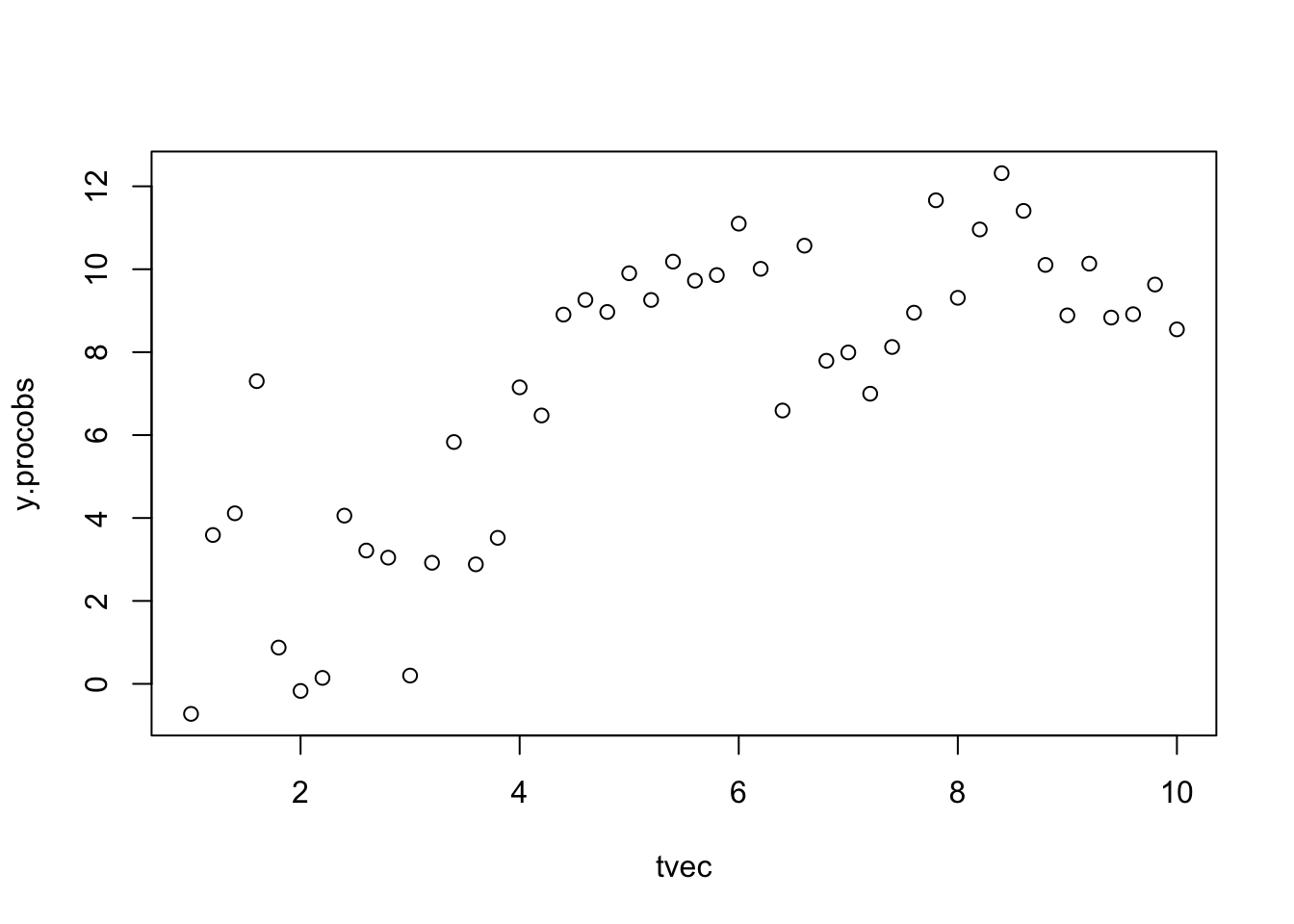I started this example to help a student implement an epidemic model where we wanted to have the dynamics (Euler steps) occurring on a faster time scale/more frequently than the observations. I ended up doing this by coding missing data/intermediate time steps as negative values and telling Stan to skip the predicted-observed comparison for those cases. (I think this is actually fairly straightforward way to deal with missing data (there is various discussion of how to model missing data in Stan, I haven’t spent long enough to see if my approach is reasonable or not).

In the process I had fun writing some “tidying” methods (i.e., for the broom package) for rstan and rjags models.

library("R2jags")
library("rstan")
options(mc.cores = parallel::detectCores())
rstan_options(auto_write = TRUE)
library("emdbook")  ## for perturb.params(): maybe unnecessary?
library("lattice")
library("plyr")
library("ggplot2"); theme_set(theme_bw())

## if necessary get broom package for tidying
## (BMB's development version has tidiers for 'rjags', 'rstan' objects)
if (FALSE) {
library("devtools")
install_github("bbolker/broom")
}
library("broom")

Simulation function:

simlogistdata <- function(seed=1001,
r=1,K=10,n0=1,t0=1,tot.t=10,dt=0.5,
sd.proc=1,sd.obs=1) {
if (!is.null(seed)) set.seed(seed)
tvec <- seq(1,tot.t,by=dt)
n <- length(tvec)
y <- numeric(n)
ydet<-numeric(n)
y <- n0
ydet <- n0
e.proc <- rnorm(n,mean=0,sd=sd.proc)
e.obs <- rnorm(n,mean=0,sd=sd.obs)
for (i in 2:n) {
ydet[i] <- ydet[i-1]+r*ydet[i-1]*(1-ydet[i-1]/K)*dt
y[i] <- y[i-1]+(r*y[i-1]*(1-y[i-1]/K))*dt
y[i] <- y[i] + e.proc[i-1]
## note we have to be careful here if we are doing anything
## non-trivial with dt; nothing is done to ensure that
## variance of e.proc scales appropriately with dt ...
}
y.procobs <- y+e.obs
return(cbind(tvec,y.procobs))
}
## now sim data ...
set.seed(102)
s1 <- simlogistdata(tot.t=10,dt=0.2)
plot(y.procobs~tvec,data=s1)JAGS preliminaries.

model {
## initial values
t <- n0
o ~ dnorm(t,tau.obs)
## step through observations
for (i in 2:N) {
v[i] <- t[i-1]+r*t[i-1]*(1-t[i-1]/K)*dt
t[i] ~ dnorm(v[i],tau.proc)
o[i] ~ dnorm(t[i],tau.obs)
}
## aux variables
sd_proc <- 1/sqrt(tau.proc)
sd_obs <- 1/sqrt(tau.obs)
## priors
r ~ dunif(0.1,maxr)
K ~ dgamma(0.005,0.005)
tau.obs ~ dgamma(0.005,0.005)
tau.proc ~ dgamma(0.005,0.005)
n0 ~ dgamma(1,n0rate)
}
parameters <- c("r","K","sd_obs","sd_proc","n0")
inits <- perturb.params(
list(n0=1,r=0.2,K=10,tau.obs=1,tau.proc=1),
alt=list(r=c(0.1,0.4),tau.obs=3,tau.proc=3))

jagsdata <- list(o=s1[,2],dt=0.2,N=nrow(s1),n0rate=1,
maxr=5)

Run JAGS:

j1 <- jags(data=jagsdata,
inits, param=parameters,
model="logist.bug",
n.chains=length(inits), n.iter=15000,
progress.bar="none",  ## quiet ...
n.burnin=5000,n.thin=37)